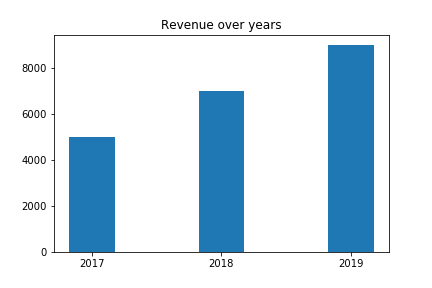## Introduction to matplotlib – Part 3

After laying the foundation in Introduction to matplotlib and Introduction to matplotlib – Part 2 I want to show you another important chart

## Bar Charts

A bar chart is useful to show total values over time e.g. the revenue of a company.

```years = (2017, 2018, 2019)
revenue = (5000, 7000, 9000)
plt.bar(years, revenue, width=0.35)
plt.xticks(years)
plt.title("Revenue over years")```Continue reading “Introduction to matplotlib – Part 3”

## Linear Regression with sklearn – cheat sheet

```# import and instantiate model
from sklearn.linear_model import LinearRegression
model = LinearRegression()

#prepare test data
features_train = df_train.loc[:, 'feature_name']
target_train = df_train.loc[:, 'target_name']

#fit (train) model and print coefficient and intercept
model.fit(features_train , target_train )
print(model.coef_)
print(model.intercept_)

# calculate model quality
from sklearn.metrics import mean_squared_error
from sklearn.metrics import r2_score

target_prediction = model.predict(features_train)
print(mean_squared_error(target_train , target_prediction))
print(r2_score(target_train , target_prediction))

# test predictions
features_test = df_train.loc[:, 'feature_name']
target_test = df_train.loc[:, 'target_name']
target_prediction_test = model.predict(features_test)
print(mean_squared_error(target_test, target_prediction_test ))
print(r2_score(target_test, target_prediction_test ))```

## pytest Tutorial – Part 1

At PyConDE Berlin 2019 Florian Bruhin gave a really nice session about testing with pytest, which I try to recap here.

## Writing Tests

If You want to work with pytest you can install it via:

`pip install pytest`

When you know basic python unittest fixtures, good news ahead:

pytest is compatible and will run your old unittest classes as well, but pytest doesn’t need to have a testclass derived from some subclass.

It is sufficient to have a single python file which name starts with test_ containing a function which name also starts with test_ Continue reading “pytest Tutorial – Part 1”